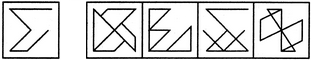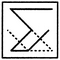# Non Verbal Reasoning - Embedded Images - Discussion

### Discussion :: Embedded Images - Section 1 (Q.No.31)

In each of the following questions, you are given a figure (X) followed by four alternative figures (1), (2), (3) and (4) such that figure (X) is embedded in one of them. Trace out the alternative figure which contains fig. (X) as its part.

31.

Find out the alternative figure which contains figure (X) as its part.(X)                (1)         (2)         (3)        (4)

 [A]. 1 [B]. 2 [C]. 3 [D]. 4

Explanation:Yadav said: (Sep 3, 2017) A is also correct.

 Soumya said: (Aug 31, 2020) A option is also correct if we rotate the (X) figure 90° in anti-clockwise direction. Moreover, (A) option seems to be more easily interpretable than (C).